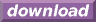#Calcute: freeware calculator

## Arccosecant (arc-cosecant, inverse cosecant, acsc)

The arc-cosecant is the inverse of the cosecant function. Calcute implements it with the acsc function. The inverse cosecant of a value x is the value y for which the cosecant of y is x. In other words if y = acsc(x) then x = csc(y). And since the cosecant takes an angle as input parameter, the arccosecant produces an angle as its output.

```acsc(1)
1.57079632679
```

The acsc value is expressed by Calcute using the currently-selected angle unit: radian, degree or gradient. In the above example, the result is in radians.

The arc-cosecant function is undefined in the open range (-1, +1); this matches the output domain of the cosecant function. Note also that the cosecant function is periodic: its values repeat infinitely for angles that represent more than one complete rotation. Conversely, the arccosecant is a multi-valued function: any parameter value matches an infinite number of possible angles. But the acsc function generates a single value refered to as the principal value. This is the "nearest" valid angle that remains within one whole revolution. All other possibilities can be obtained by adding or subtracting any whole number of rotations from the return value of acsc.

In some mathematical texts and hand-held calculators, the notation csc-1 is sometimes used to represent the arc-cosecant function. This is an unfortunate notational choice since it could also stand for the multiplicative inverse 1/csc, which is a different function.

Yet another term also used to represent the inverse cosecant function is "arccsc".